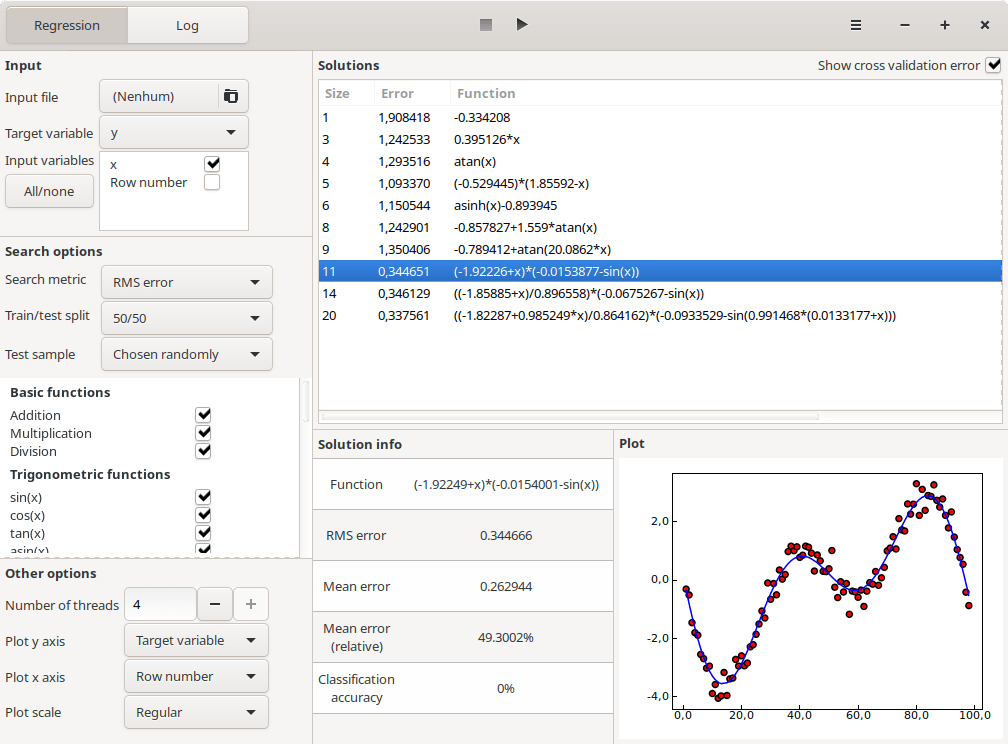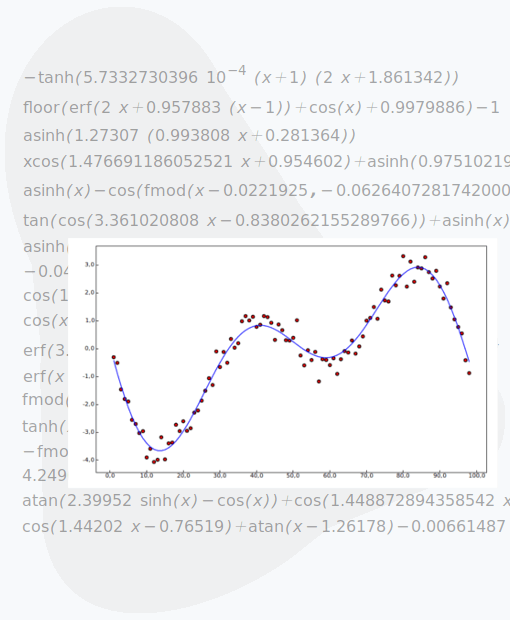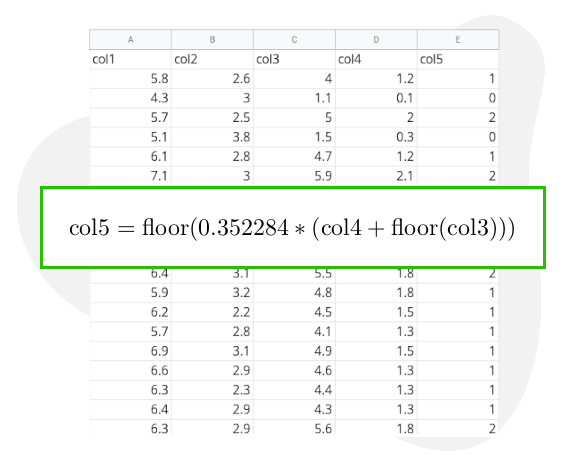## A new way of doing machine learning.

TuringBot finds mathematical formulas that connect variables, uncovering hidden relationships. Trusted by engineers and researchers around the world.

## Features

The software is based on a technique called Symbolic Regression.
Here is what it can do:### Regression and classification

Multiple built-in error metrics for the optimization are included, allowing different kinds of models to be generated. They include:

• Root mean square error, for regression problems;
• Classification accuracy, for classification problems (by representing categorical variables as different integer numbers);
• F1 score, for predicting rare events (classification problems where the label is 0 most of the time, and 1 in some relevant cases).### Machine learning without black boxes

Symbolic models are transparent: they show exactly which variables are being used and how. This allows invaluable insight to be gained into the data that is being modeled.

TuringBot shows that an AI model does not need to be a neural network with hundreds of weights. It can be simple and just as effective.### Pareto optimization

TuringBot simultaneously optimizes for formulas of all possible sizes. It will give you not only a single mathematical expression, but a set of expressions of increasing complexity to choose from.

The optional cross validation feature can be activated to help select the formula with the best trade off between complexity and accuracy, allowing overfit solutions to be ruled out in a straightforward way.